## “A very general approach to train Neural Networks is to use Gradient Descent Algorithm and other variants of it, but this requires the prior information of gradient in the search space. The gradient information may not be available or may not be very feasible for some problems. Evolutionary algorithms provide a pool of algorithms to train neural networks or find optimal points in a search space without requiring the gradient information.”

Conventional gradient descent algorithm uses the update step for training neural networks for different problems which can be represented as below: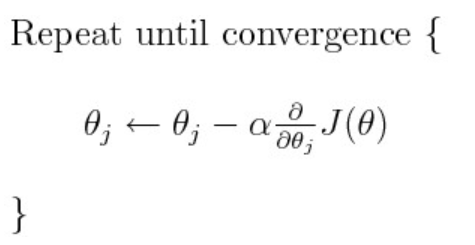Here, J(θ) is the cost function and in each iteration, parameter θj is updated as in the equation above. This will continue iteratively until the cost function is minimized. One important aspect in this algorithm is that we need to calculate the gradient for each update step. In this article we will try to introduce evolutionary methods and algorithms which can be used to train neural networks without requiring to know the gradient information beforehand.

In Computer Science, the ability of a computer to learn a specific task from data or experimental observation is referred to as Computational Intelligence (CI). CI comprises many computational techniques and Evolutionary Computing is one of its parts. It is a family of algorithms for global optimization inspired by biological evolution occurring in nature, and the subfield of artificial intelligence and soft computing studying these algorithms. In technical terms, they are a family of population-based trial and error problem solvers with a metaheuristics or stochastic optimization character.

What do we mean by a metaheuristic? In computer science, artificial intelligence, and mathematical optimization, a heuristic is a technique designed for solving a problem more quickly when classic methods are too slow, or for finding an approximate solution when classic methods fail to find any exact solution. A metaheuristic is defined as an iterative approach which guides a subordinate heuristic by introducing different concepts for exploring and exploiting search space in an intelligent way. They are inspired by observing the phenomena occurring in nature. As an example one may take an intuition from the evolution of human beings, the way humans have evolved over ages by expressing dominant genes over many evolutions of time. Metaheuristics sample a subset of solutions which is otherwise too large to be completely enumerated or otherwise explored. Metaheuristics may make relatively few assumptions about the optimization problem being solved and so may be usable for a variety of problems.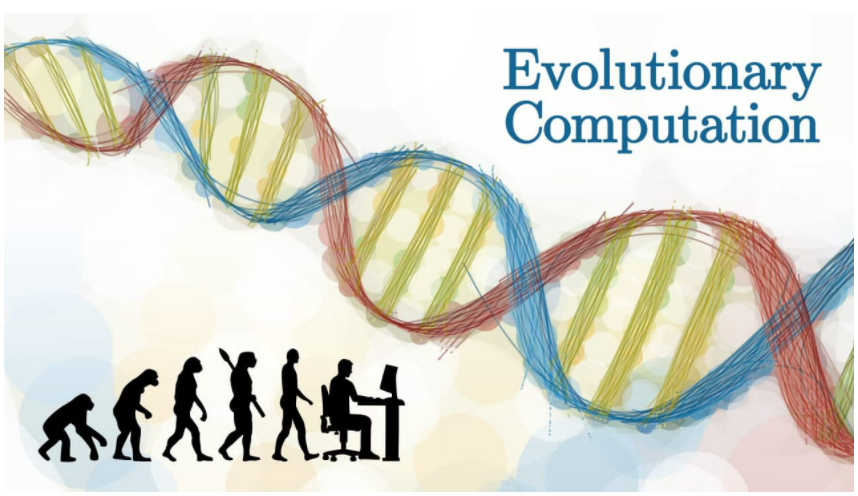How do we define an Evolutionary Algorithm? – An evolutionary algorithm (EA) is a subset of the family of evolutionary computation, a generic population-based metaheuristic optimization algorithm. An EA uses mechanisms inspired by biological evolution, such as reproduction, mutation, recombination, and selection. Candidate solutions to the optimization problem play the role of individuals in a population, and the fitness function(Judging Parameter for a Candidate Solution) determines the quality of the solutions. Evolution of the population then takes place after the repeated application of the above operators.

How do we define our problem? – We will generally define the problem as: We wish to find the best combination of elements that minimize some fitness function, and we will accept a final solution once we have either ran the algorithm for some maximum number of iterations, or we have reached some fitness threshold. This scenario is clearly not the only way to use an EA, but it does encompass many common applications in the discrete case.

Different Steps involved in an Evolutionary Algorithm can be represented as below: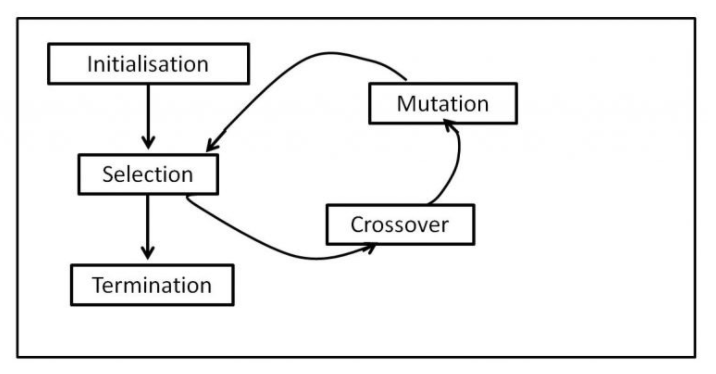• Initialization:

In order to begin our algorithm, we must first create an initial population of solutions. The population will contain an arbitrary number of possible solutions to the problem, often called as candidate solutions (particles or genes). It will often be created randomly within the constraints of the problem or if some prior knowledge of the task is known, roughly centered around what is believed to be ideal. It is important that the population encompasses a wide range of solutions, because it essentially represents a pool of genes and we wish to explore many different possibilities over the course of the algorithm, we should aim to have many different genes present.

• Selection:

Once a population is created, candidate solutions in the population must now be evaluated according to a fitness function. A fitness function is a function that takes in the characteristics of a solution, evaluates it and outputs a numerical representation of how important a solution it is. If we take intuition from Neural Networks we want to minimize the Loss Function(example: RMSE, Cross-Entropy Loss etc.) and one can always correspond this loss as the fitness function and we want to minimize it i.e a solution having lower fitness value is more important. Creating the fitness function can often be very difficult, and it is important to find a good function that accurately represents the data; it is very problem-specific. Now, we calculate the fitness of all members, and select a portion of the top-scoring candidate solutions.

• Mutation:

Inspired by the role of mutation of an organism’s DNA in natural evolution an evolutionary algorithm periodically makes random changes or mutations in one or more members of the current population, yielding a new candidate solution which may be better or worse than existing population members. Different evolutionary algorithms differ in how they produce mutations in candidate solutions. The result of a mutation may be an infeasible solution, and the Evolutionary Algorithm attempts to “repair” or “improve” such a solution to make it feasible; this is sometimes, but not always, successful.

• Cross-Over:

As cited earlier the evolutionary algorithms take inspiration by the role of sexual reproduction in the evolution of living things and attempts to combine elements of existing solutions in order to create a new solution with some of the features of previous generation (parents). The elements of existing solutions are combined in a “crossover” operation, inspired by the crossover of DNA strands that occurs in reproduction of biological organisms. As with mutation, there are many possible ways to perform a crossover operation, some feasible and some not, and the Evolutionary Algorithm actually employs multiple variations of different crossover strategies.

After one cycle is completed, an evolutionary algorithm performs the selection process (selection step) in which the “most fit” members of the population survive, and the “least fit” members are eliminated. The selection process is the step that guides the evolutionary algorithm towards ever-better solutions. This cycle is repeated unless and until we get a solution with a particular threshold fitness value.

Till this point we have a good basic knowledge about the concepts involved in an Evolutionary Algorithm and now we will try to understand it with an example. There are different Evolutionary Algorithms available in the literature such as PSO, Differential Evolution, Evolution Strategies, etc and one may try them depending on the problem in hand as well as the resources available.

We will try to understand the basic implementation of PSO (Particle Swarm Optimization) by first learning its theoretical concepts and then we will hop onto its example code.

Particle Swarm Optimization (PSO): It is an evolutionary algorithm that optimizes a problem by iteratively trying to improve a candidate solution with regard to the fitness values of multiple candidate solutions. It solves a problem by having a population of candidate solutions. In PSO, the population of candidate solutions is known as swarm and each candidate solution in the population is known as a particle. So basically, a swarm is composed of multiple particles. The particles get updated in the search-space according to the position and velocity of the particle where each particle’s movement depends on its local best known position and is also guided toward the best known positions in the swarm. Subsequently, update is performed as better positions are found by other particles.

An example simulation of PSO can be seen below:While updating the particles in the swarm, three different components are taken into account:

1. Physical (or Inertial) component which allows the particle to follow its own path of displacement.

2. Social component which allows the particle to follow the global best particle in the swarm.

3. Cognitive component which allows the particle to get attracted and follow its personal best position found till a particular number of iterations.

PSO Update Equations:

Each particle can be represented by a position xi=(xi1,xi2,…,xiN) and a velocity vi=(vi1,vi2,…,viN) in the N-dimensional problem space, where i denotes the ith particle and N represents the dimension of the problem or number of unknown variables. PSO is initialized with a group of random particles (candidate solutions) and then search is performed by updating these particles over generations to find a possible optimum in the search space. During every iteration (or generation), each particle is updated by following two important terms. The first one is the position vector of the best solution (fitness) this particle has achieved so far which is also stored. This position is called pbest. Another position is the best position obtained so far by any particle in the population. This best position is the current global best and is called gbest. After finding the two best values, the position and velocity of the particles are updated by the following two equations: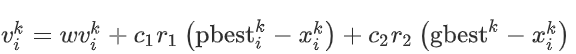–(1)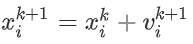–(2)

where vik is the velocity of the ith particle at the kth iteration, and xik is the current solution (or position) of the ith particle at the kth iteration. c1,c2 are positive constants, and r1, r2 are two random variables with uniform distribution between 0 and 1. In this equation, w is the inertia weight which shows the effect of the previous velocity vector on the new vector. An upper bound(max) as well as lower bound(min) is placed on the velocity in all dimensions. This limitation prevents the particle from moving too rapidly from one region in the search space to another. This value is usually initialized as a function of the range of the problem. In equation (1), the first term is the inertia term, second is the cognitive term and the third is the social term. Updates are performed using these update terms for each particle in each iteration unless and until we find a solution having fitness value below a particular threshold.

Code:

As cited earlier, we can use PSO as an evolutionary algorithm to train neural networks of different sizes. As an example to simulate the optimization behaviour of PSO, we consider the McCormick function to simulate the search space produced by a neural network (This is just for an example representation).

McCormick Function is represented below: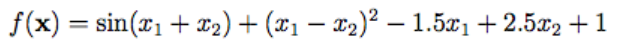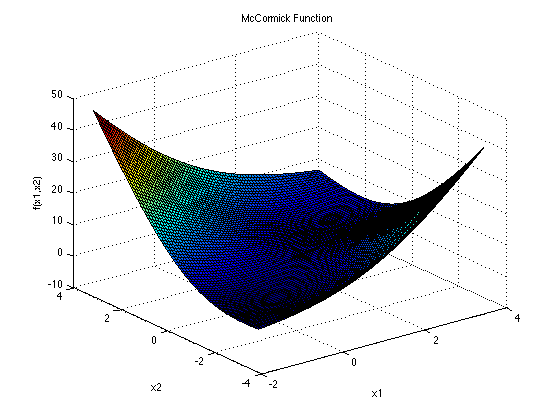//Important keyword to run the code on s2
%use s2


				
import java.util.* // Accessing all classes and methods in Java
import kotlin.jvm.JvmStatic// Specifies that an additional static method needs to be generated from this element if this is
// a function
import java.util.Random // To generate pseudo-random numbers


				
typealias Func = (DoubleArray) -&gt; Double

class Parameters(val omega: Double, val phip: Double, val phig: Double)//A class for instatiating an object which specifies
//the three important parameters used in PSO.

class State(
val iter: Int, // Number of iterations completed
val gbpos: DoubleArray, // Global Best Position
val gbval: Double, // Global Best Value
val min: DoubleArray, // The lower limit on the value of particles
val max: DoubleArray, // The Upper limit on the value of particles
val parameters: Parameters, // Parameters used in PSO
val pos: Array, // Specifies the current position of the particle
val vel: Array, // Specifies the current value of the particle
val bpos: Array, // Specifies the best position of the particle in the flock
val bval: DoubleArray, // Specifies the best value of the particle in the flock
val nParticles: Int, // number of particles in the flock
val nDims: Int // Number of Dimensions
) {
fun report(testfunc: String) { // Printing the report
println("Test Function : $testfunc") println("Iterations Completed :$iter")
println("Global Best Position found using PSO: ${gbpos.asList()}") println("Global Best Value found using PSO:$gbval")
}
}


				
// Function used to initialize the particles in the flock before starting the evolution.
fun psoInit(
min: DoubleArray, // the array of size (1,dimension) and all the values are min(lower bound)
max: DoubleArray, // the array of size (1,dimension) and all the values are max(Upper bound)
parameters: Parameters,
nParticles: Int
): State {
// Initializing different variables used in PSO.
val nDims = min.size
val pos   = Array(nParticles) { min }
val vel   = Array(nParticles) { DoubleArray(nDims) }
val bpos  = Array(nParticles) { min }
val bval  = DoubleArray(nParticles) { Double.POSITIVE_INFINITY}
val iter  = 0
val gbpos = DoubleArray(nDims) { Double.POSITIVE_INFINITY }
val gbval = Double.POSITIVE_INFINITY
return State(iter, gbpos, gbval, min, max, parameters,
pos, vel, bpos, bval, nParticles, nDims)
}

val r = Random() // random variable


				
// Main function involving evolution of the flock of particles using PSO
fun pso(fn: Func, y: State): State {
val p = y.parameters
val v = DoubleArray(y.nParticles)
val bpos  = Array(y.nParticles) { y.min }
val bval  = DoubleArray(y.nParticles)
var gbpos = DoubleArray(y.nDims)
var gbval = Double.POSITIVE_INFINITY
// Here, for each particle in the flock we update the best position as well as value for the particle
// if the newly found value is a better one, we also update the global best position and value using the updated values of
// particles.
for (j in 0 until y.nParticles) {
// evaluate
v[j] = fn(y.pos[j])
// update
if (v[j] &lt; y.bval[j]) { // if newly found value is lesser(improved) then update best position and value to the current ones.
bpos[j] = y.pos[j]
bval[j] = v[j]
}
else {
bpos[j] = y.bpos[j]// else re-assign it to the previously found best ones.
bval[j] = y.bval[j]
}
if (bval[j] &lt; gbval) {// if value of current particle is smaller than the global value found previously, update it.
gbval = bval[j]
gbpos = bpos[j]
}
}
val rg = r.nextDouble()
val pos = Array(y.nParticles) { DoubleArray(y.nDims) }
val vel = Array(y.nParticles) { DoubleArray(y.nDims) }
for (j in 0 until y.nParticles) { // In this loop we try to update the position of all the particles in the flock
// migrate
val rp = r.nextDouble()
var ok = true // variable to check if all dimension values of a particle fall in range [lower bound,upper bound] or not.
vel[j].fill(0.0)
pos[j].fill(0.0)
for (k in 0 until y.nDims) {// For each dimension of a particle(In our example case it is only two)
vel[j][k] = p.omega * y.vel[j][k] +
p.phip * rp * (bpos[j][k] - y.pos[j][k]) +
p.phig * rg * (gbpos[k] - y.pos[j][k]) // Velocity update formula
pos[j][k] = y.pos[j][k] + vel[j][k] // Position update formula
ok = ok &amp;&amp; y.min[k]  pos[j][k] // ok will be set as false if range [lower bound,upper bound] is not satisfied.
}
if (!ok) { // If found false then re-initialize the position of current particle
for (k in 0 until y.nDims) {
pos[j][k]= y.min[k] + (y.max[k] - y.min[k]) * r.nextDouble()
}
}
}
val iter = 1 + y.iter // Increase the number of iterations.
return State(
iter, gbpos, gbval, y.min, y.max, y.parameters,
pos, vel, bpos, bval, y.nParticles, y.nDims
)
}


				
// A simple function to perform required number of iterations using PSO.
fun iterate(fn: Func, n: Int, y: State): State {
var r = y
var old = y
if (n == Int.MAX_VALUE) {
while (true) {
r = pso(fn, r)
if (r == old) break
old = r
}
}
else {
repeat(n) { r = pso(fn, r) }
}
return r
}

// Defining Mccormic Function
fun mccormick(x: DoubleArray): Double {
val (a, b) = x
return Math.sin(a + b) + (a - b) * (a - b) + 1.0 + 2.5 * b - 1.5 * a
}



				
// defining different variables which we will use for our example code
var state = psoInit(
min = doubleArrayOf(-1.5, -3.0), // lower bound
max = doubleArrayOf(4.0, 4.0), // upper bound
parameters = Parameters(0.1, 0.6, 0.3), // (omega , c1 , c2)
nParticles = 100 // number of candidate particles
)
var number_of_iterations = 30 // Number of iterations of evolution required using PSO
state = iterate(::mccormick, number_of_iterations, state)
state.report("McCormick")
// Theoretical global minimum value of mccormick function is found at position (-.54719, -1.54719)
println("f(-0.54719, -1.54719) : \${mccormick(doubleArrayOf(-.54719, -1.54719))}")



Output: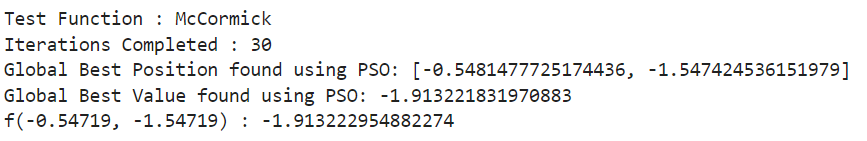We can see that in 30 iterations of evolution using PSO we were almost able to reach the theoretical global minimum value of the McCormick Function
which occurs at position (-0.54719, -1.54719).

Limitations:

• Although Evolutionary Algorithms don’t require gradient information for optimization, each iteration requires updating each particle present in the population which makes it a very computationally expensive approach to optimization as compared to gradient descent. So training bigger neural networks using Evolutionary Algorithms will take way more time as compared to Gradient Descent Algorithm.

• Another limitation of any evolutionary algorithm is that a solution is “better” only in comparison to other candidate solutions in the population, there is no particular concept of a “global optimal solution”. Although in our example code for optimizing McCormick function we were able to almost reach the global minimum as it appears to be an easy search space to explore for PSO, evolutionary algorithms are prone to be stuck in local minimum points for other difficult problems. For this reason, evolutionary algorithms are best employed on problems where it is difficult to test for optimal behaviour. This also means that an evolutionary algorithm never knows for certain when to stop, aside from the length of time or the number of iterations that you wish to allow it to explore.